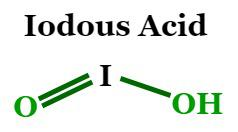Open in App
Not now

# Iodous Acid Formula

• Last Updated : 01 Nov, 2022

Iodous acid is an iodine oxoacid and is also known as hydroiodic acid. Its chemical formula is HIO2, and it is the conjugate acid of an iodite. It is extremely unstable and can only be present briefly in aqueous solutions. Its molecular formula is 159.911 g/mol, while its monoisotopic mass is 159.90213 g/mol. An iodite ion, or iodine dioxide anion, is called halite. The oxidation number of iodine in iodous acid is +3. The salts of iodous acid are known as iodites and are observed, but they are very unstable and are never isolated. These salts are disproportionate and quickly form molecular iodates and iodine. The hydrogen bond donor count in iodous acid is 1, while its hydrogen bond acceptor count is 2.

## Structure Of Iodous acid

The chemical formula of iodous acid is HIO2. It is made up of a hydrogen atom, an iodine atom, and two oxygen atoms. An iodine atom is bonded to an oxygen atom and a hydroxyl group. The two structural isomers of HIO2 are HOOI and HOIO, where H, O, and I atoms are arranged differently within the molecules.## Preparation Of Iodous acid

HOIO can be produced from IO + OH and I + HO2 reactions. Despite the significance of iodous acid (HOIO) in nuclear and atmospheric chemistry, computational research on HIO2 species is rare, and experimental proof of iodous acid in the gas phase has just recently been documented in the literature.

IO + OH → HOIO

I + HO2 → HOIO

## Chemical Properties of Iodous acid

• When iodous acid reacts with sodium hydroxide, sodium iodite (NaIO2) is formed.

HIO2 + NaOH → H2O + NaIO2

• Zinc iodite (Zn(IO2)2) is formed when iodous acid reacts with zinc hydroxide.

HIO2 + Zn(OH)2 → Zn(IO2)2 + H2O

• When iodous acid reacts with hydrogen iodide to give hydrogen peroxide and iodine.

HI + HIO2 → H2O2 + I2

## FAQs on Iodous Acid

Question 1: What is the chemical formula of Iodous Acid?

The chemical formula of iodous acid is HIO2. From its chemical formula, we can say that it is made up of a hydrogen atom, an iodine atom, and two oxygen atoms.

Question 2: Calculate the molecular mass of iodous acid.

We know that the chemical formula of iodous acid is HIO2.

Atomic mass of a hydrogen atom = 1.00794

Atomic mass of an iodine atom = 126.90447

Atomic mass of an oxygen atom = 15.9994

Now, the molecular of iodous acid = 1.00794 + 126.90447 + 2 × 15.9994

= 1.00794 + 126.90447 + 31.9988 = 159.91121 g/mol

So, the molecular mass of iodous acid is 159.91121 g/mol.

Question 3: Mention some properties of Iodous Acid.

The chemical formula of iodous acid is HIO2. Its molar mass is 159.911 g/mol and it has a complexity of 10.3. The hydrogen bond donor count in Iodous Acid is 1, while its hydrogen bond acceptor count is 2.

Question 4: What happens when Iodous Acid reacts with Sodium Hydroxide?

When iodous acid reacts with sodium hydroxide, sodium iodite (NaIO2) is formed.

HIO2 + NaOH → H2O + NaIO2

Question 5: What happens when Iodous Acid reacts with Hydrogen Iodide?